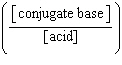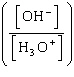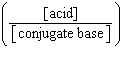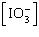Name:    chapter 17 online practice

Multiple Choice
Identify the letter of the choice that best completes the statement or answers the question.

1.

What is the effect of adding 10 mL of 0.1 M NaOH(aq) to 100 mL of 0.2 M NH4+(aq)?

 1. The pH will decrease. 2. The concentration of NH3 will increase. 3. The concentration of NH4+ will decrease.
 a. 1 only b. 2 only c. 3 only d. 2 and 3 e. 1, 2, and 3

2.

What is the pH of a solution that results from adding 25 mL of 0.15 M HCl to 25 mL of 0.52 M NH3? (Kb of NH3 = 1.8 ´ 10-5)
 a. 2.74 b. 4.35 c. 9.65 d. 11.26 e. 11.41

3.

Which of the following combinations would be best to buffer an aqueous solution at a pH of 2.0?
 a. H3PO4 and H2PO4-, Ka1 = 7.5 ´ 10-3 b. HNO2 and NO2-, Ka = 4.5 ´ 10-4 c. CH3CO2H and CH3COO-, Ka = 1.8 ´ 10-5 d. H2PO4- and HPO42-, Ka2 = 6.2 ´ 10-8 e. NH4+ and NH3, Ka = 5.7 ´ 10-10

4.

Which of the following combinations would be the best to buffer an aqueous solution at a pH of 5.0?
 a. H3PO4 and H2PO4-, Ka1 = 7.5 ´ 10-3 b. HNO2 and NO2-, Ka = 4.5 ´ 10-4 c. CH3CO2H and CH3COO-, Ka = 1.8 ´ 10-5 d. H2PO4- and HPO42-, Ka2 = 6.2 ´ 10-8 e. NH4+ and NH3, Ka = 5.7 ´ 10-10

5.

Each of the following mixtures can produce an effect buffer solution EXCEPT
 a. HClO4 and NaClO4. b. HF and NaF. c. NaHCO3 and Na2CO3. d. Na2HPO4 and Na3PO4. e. NH4Cl and NH3.

6.

All of the following statements concerning buffers are true EXCEPT
 a. buffers are resistant pH changes upon addition of small quantities of strong acids or bases. b. buffers are used as colored indicators in acid-base titrations. c. the pH of a buffer is close to the pKa of the weak acid from which it is made. d. buffers contain appreciable quantities of a weak acid and its conjugate base. e. buffers are resistant to changes in pH when diluted with water.

7.

Which of the following mathematical expressions is the Henderson-Hasselbalch equation?
 a. pKa = pH + logb. pH = pKa + logc. pH = pKa + logd. pKa = pH - loge. pH = pKa + log8.

What is the pH of the buffer that results when 4.0 g of NH3 and 8.0 g of NH4Cl are diluted with water to a volume of 0.50 L? (Ka of NH4+ = 5.6 ´ 10-10)
 a. 8.95 b. 9.06 c. 9.25 d. 9.45 e. 9.55

9.

What mass of solid KF (molar mass = 58.1 g/mol) should be added to 2.0 L of 0.25 M HF to make a buffer with a pH of 3.14? (pKa for HF = 3.14)
 a. 7.3 g b. 15 g c. 29 g d. 58 g e. 120 g

10.

If the ratio of acid to base in a buffer increases by a factor of 10, the pH of the buffer
 a. decreases by 1. b. decreases by 10. c. increases by 10. d. increases by 1. e. remains unchanged.

11.

A buffer is prepared by combining 250 mL of 0.25 M NaOH and 250 mL of a 0.600 M weak acid, HA. If the pH of the buffer is 6.33, what is the pKa of the acid?
 a. 5.95 b. 6.18 c. 6.33 d. 6.48 e. 6.71

12.

How many moles of HCl must be added to 1.00 L of 0.72 M NH3 to make a buffer with a pH of 9.50? (Ka of NH4+ = 5.6 ´ 10-10)
 a. 0.26 mol b. 0.31 mol c. 0.41 mol d. 0.46 mol e. 1.3 mol

13.

Which one of the following conditions is always met at the equivalence point of the titration of a monoprotic weak base with a strong acid?
 a. The pH of the solution is equal to 7.00. b. The volume of acid added from the buret equals the volume of base titrated. c. The molarity of the acid equals the initial molarity of the weak base. d. The percent ionization of the acid equals the percent ionization of the base. e. The moles of acid added from the buret equals the initial moles of weak base.

14.

Which one of the following conditions is always true for a titration of a weak acid with a strong base?
 a. A colored indicator with a pKa less than 7 should be used. b. If a colored indicator is used, it must change color rapidly in the weak acid's buffer region. c. Equal volumes of weak acid and strong base are required to reach the equivalence point. d. The equivalence point occurs at a pH equal to 7. e. The equivalence point occurs at a pH greater than 7.

15.

A volume of 25.0 mL of 0.100 M HCO2H(aq) is titrated with 0.100 M NaOH(aq). What is the pH after the addition of 12.5 mL of NaOH? (Ka for HCO2H = 1.8 ´ 10-4)
 a. 2.52 b. 3.74 c. 4.74 d. 7.00 e. 10.26

16.

A 25.0 mL sample of 0.0200 M NH3(aq) is titrated with 0.0100 M HCl(aq). What is the pH at the equivalence point? (Kb of NH3 = 1.8 ´ 10-5)
 a. 3.46 b. 5.48 c. 5.72 d. 8.25 e. 10.54

17.

A 50.0 mL sample of 0.0240 M NH3(aq) is titrated with aqueous hydrochloric acid. What is the pH after the addition of 15.0 mL of 0.0600 M HCl(aq)? (Kb of NH3 = 1.8 ´ 10-5)
 a. 8.78 b. 8.86 c. 9.25 d. 9.38 e. 9.73

18.

Potassium hydrogen phthalate (molar mass = 204.2 g/mol) is used to standardize sodium hydroxide. If 26.37 mL of NaOH(aq) is required to titrate 0.7719 g KHP to the equivalence point, what is the concentration of the NaOH(aq)?

HC8H4O4-(aq) + OH-(aq) f C8H4O42-(aq) + H2O(l)
 a. 0.02036 M b. 0.02937 M c. 0.09968 M d. 0.1433 M e. 5.977 M

19.

A 50.0 mL sample of vinegar is titrated with 0.774 M NaOH(aq). If the titration requires 41.6 mL of NaOH(aq), what is the concentration of acetic acid in the vinegar?
 a. 0.0921 M b. 0.429 M c. 0.644 M d. 0.930 M e. 0.967 M

20.

An impure sample of sodium carbonate, Na2CO3, is titrated with 0.150 M HCl according to the reaction below.

2 HCl(aq) + Na2CO3(aq) f CO2(g) + H2O(l) + 2 NaCl(aq)

What is the percent of Na2CO3 in a 0.927 g sample if the titration requires 27.3 mL of HCl? The molar mass of Na2CO3 is 106.0 g/mol.
 a. 0.221% b. 23.4% c. 46.8% d. 93.7% e. 104%

21.

Which is the best colored indicator to use in the titration of 0.0010 M CH3CO2-(aq) with HCl(aq)? Why? (Kb of CH3CO2- = 5.6 ´ 10-10)

 Indicator p K a Bromocresol green 4.7 Phenol Red 7.8 Phenolphthalein 9.0
 a. Bromocresol green. The pH at the equivalence point is less than 7.0. b. Phenol Red. The pKb of acetate ion and the pKb of the indicator are similar. c. Phenol Red. The equivalence point of an acid-base titration occurs at a pH of 7.0. d. Phenolphthalein. The pKb of acetate ion and the pKb of the indicator are similar. e. Phenolphthalein. The pH at the equivalence point is greater than 7.0.

22.

What color change is exhibited by phenolphthalein during a titration of aqueous acetic acid with aqueous sodium hydroxide?
 a. colorless to pink b. pink to colorless c. green to yellow d. yellow to blue e. blue to yellow

23.

Which of the following equations is the solubility product for magnesium iodate, Mg(IO3)2?
 a. Ksp = [Mg2+][I-]2[O2-]6 b. Ksp = [Mg2+][I-]2[3O2-]2 c. Ksp = [Mg2+]d. Ksp = [Mg2+]2e. Ksp = [Mg2+]2

24.

The solubility of SrSO4 in water is 0.107 g in 1.0 L at 25 °C. What is the value of Ksp for SrSO4?
 a. 3.4 ´ 10-7 b. 5.8 ´ 10-4 c. 1.2 ´ 10-3 d. 1.1 ´ 10-2 e. 2.1 ´ 10-1

25.

The following anions can be separated by precipitation as silver salts: Cl-, Br-, I-, CrO42-. If Ag+ is added to a solution containing the four anions, each at a concentration of 0.10 M, in what order will they precipitate?

 Compound K sp AgCl 1.8 ´ 10 -10 Ag 2 CrO 4 1.1 ´ 10-12 AgBr 5.4 ´ 10 -13 AgI 8.5 ´ 10 -17
 a. AgCl ® Ag2CrO4 ® AgBr ® AgI b. AgI ® AgBr ® Ag2CrO4 ® AgCl c. Ag2CrO4 ® AgCl ® AgBr ® AgI d. Ag2CrO4 ® AgI ® AgBr ® AgCl e. AgI ® AgBr ® AgCl ® Ag2CrO4